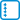## Sums of series

Sum up the following series by using the generating functions:

• #### Variant

$$\displaystyle \sum_{k=0}^n \binom{n}{k}^2$$

• #### Variant

$$\displaystyle \sum_{k=0}^n (-1)^k{n \choose k}^2$$

• #### Variant

$$\displaystyle \sum_{k=0}^n k\cdot 2^k$$

• #### Variant

$$\displaystyle \sum_{k=0}^n k {n \choose k}$$

• #### Variant

$$\displaystyle \sum_{k=0}^n k^2 \binom{n}{k}$$